# PHP Variable Scope

You can declare your variable anywhere in the PHP script. The scope of a variable is the part of that script where the variable can be referenced or used. There are following four types of variable scopes available in PHP:

• local scope
• global scope
• static scope

## PHP Local Scope

In PHP, local variables are those variables that are declared within a function. These variables has Local scope and you can only access to these variables, within that function, where they are declared. Here is an example showing local scope of a variable in PHP:

```<!DOCTYPE html>
<html>
<body>

<?php
function myTest() {
\$x = 50; // local scope
echo "<p>Variable x inside function is: \$x</p>";
}
myTest();

// using x outside the function will generate an error
echo "<p>Variable x outside function is: \$x</p>";
?>

</body>
</html>```

Here is the output produced by the above PHP script: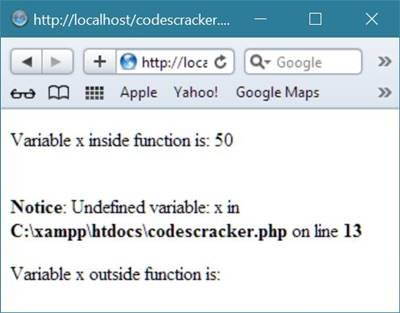Note - You can have local variables in PHP, with same name in different functions, as local variables are only recognized by that function where they are declared.

## PHP Local Variable Scope Example

Here is an example that demonstrates about the local variable scope in PHP

```<html>
<head>
<title>PHP Variable Scope - CodesCracker</title>
</head>
<body>
<?php
# PHP Variable Scope Example - CodesCracker
function fun1()
{
// both variable are local variable
\$local_variable1 = "this is local variable";
\$local_variable2 = 5;
echo \$local_variable1;
echo "<br/>";
echo \$local_variable2;
}
// call the function to run its content
fun1();
echo "<hr/>";
// will produce an error message
echo \$local_variable1;
echo "<br/>";
// will produce an error message
echo \$local_variable2;
echo "<br/>";
?>
</body>
</html>```

Here is the sample output of the above PHP local variable scope example code:## PHP Global Scope

PHP global variable are those variables, which are declared outside the function. These variables has global scope, and you can only access to these variables, outside a function. Here is an example:

```<!DOCTYPE html>
<html>
<body>

<?php
\$x = 50; // global scope

function myTest() {
// using x inside this function will generate an error
echo "<p>Variable x, inside function is: \$x</p>";
}
myTest();

echo "<p>Variable x, outside function is: \$x</p>";
?>

</body>
</html>```

Here is the output of the above PHP script: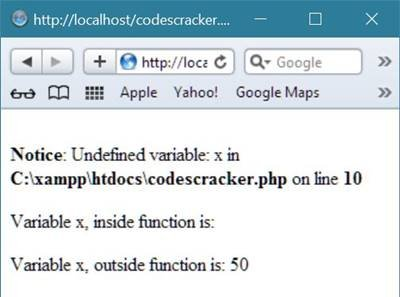## PHP Global Variable Scope Example

Let's take an example demonstrating the global scope of a variable in PHP.

```<html>
<head>
<title>PHP Variable Scope (Global) - CodesCracker</title>
</head>
<body>
<?php
# PHP Variable Scope (Global) Example - CodesCracker
// both variable are global variable with global scope
\$global_variable1 = "CodesCracker";
\$global_variable2 = 5;
function fun()
{
// above declared two global variables does not
// exist here
echo \$global_variable1;
echo "<br/>";
echo \$global_variable2;
}
fun();
echo "<hr/>";
// above declared two global variables
// exists here
echo \$global_variable1;
echo "<br/>";
echo \$global_variable2;
?>
</body>
</html>```

Here is the sample output produced by the above global variable scope example code in PHP: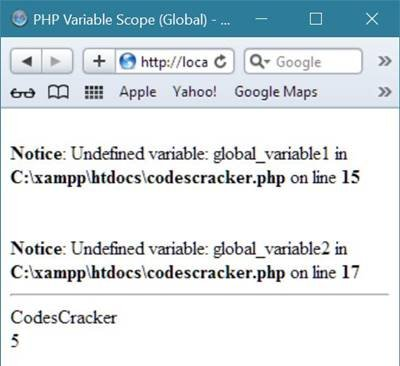## PHP global Keyword

PHP global keyword is basically used to access the global variable from within a function. Here is an example, accessing global variables in PHP using the global keyword:

```<!DOCTYPE html>
<html>
<body>

<?php
\$x = 50;
\$y = 100;

function myTest() {
global \$x, \$y;
\$y = \$x + \$y;
}

myTest(); // run function
echo \$y; // output the new value for variable \$y
?>

</body>
</html>```

Below is the output of this PHP script: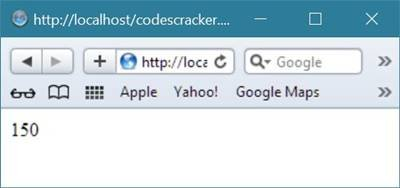Let's take another example of global keyword in PHP.

```<html>
<head>
<title>PHP global Keyword - CodesCracker</title>
</head>
<body>
<?php
# PHP global Keyword Example - CodesCracker
\$global_variable = "CodesCracker in global variable";
function fun()
{
\$local_variable = "CodesCracker in local variable";
echo \$local_variable;
echo "<br/>";
global \$local_variable_globalkey;
\$local_variable_globalkey = "CodesCracker in local variable with global keyword";
}
fun();
echo "<hr/>";
echo \$global_variable;
echo "<br/>";
echo \$local_variable_globalkey;
?>
</body>
</html>```

The above global keyword example code in PHP will produce the following output:PHP also stores all the global variables in the array named \$GLOBALS[index]. Here, the index holds the variable's name. You can also access to these array from within functions and can be used to update the global variables directly. Here is an example. This is the rewritten script of the above PHP script:

```<!DOCTYPE html>
<html>
<body>

<?php
\$x = 50;
\$y = 100;

function myTest() {
\$GLOBALS['y'] = \$GLOBALS['x'] + \$GLOBALS['y'];
}

myTest();
echo \$y;
?>

</body>
</html>```

Here is the output you will watch on your browser after running the above PHP script:## PHP static Keyword

PHP static keyword is used to hold the variable's value for further use. Here is an example, uses static keyword:

```<!DOCTYPE html>
<html>
<body>

<?php
function myTest() {
static \$x = 10;
echo \$x;
\$x++;
}

myTest();
echo "<br>";
myTest();
echo "<br>";
myTest()
?>

</body>
</html>```

Here is the output produced by this PHP script:Let's take another example demonstrating static keyword in PHP.

```<html>
<head>
<title>PHP static Keyword - CodesCracker</title>
</head>
<body>
<?php
# PHP static Keyword Example - CodesCracker
static \$static_num=0;
\$dynamic_num = 0;
function fun()
{
static \$static_num = 0;
echo \$static_num;
echo " - ";
\$static_num++;
\$dynamic_num = 0;
echo \$dynamic_num;
\$dynamic_num++;
echo "<hr/>";
}
for(\$i=0; \$i<5; \$i++)
{
fun();
}
?>
</body>
</html>```

Here is the sample output of the above static keyword example code in PHP: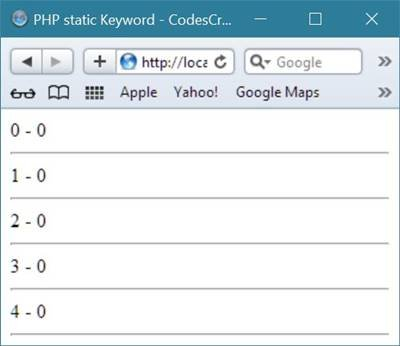Tools
Calculator

Quick Links
Signup - Login - Give Online Test